SLOS313C December   2000  – March 2016

PRODUCTION DATA.

1. Features
2. Applications
3. Description
4. Revision History
5. Device Comparison Table
6. Pin Configuration and Functions
7. Specifications
8. Parameter Measurement Information
9. Detailed Description
10. 10Application and Implementation
1. 10.1 Application Information
2. 10.2 Typical Application
11. 11Power Supply Recommendations
12. 12Layout
13. 13Device and Documentation Support
14. 14Mechanical, Packaging, and Orderable Information

#### Package Options

Refer to the PDF data sheet for device specific package drawings

• D|8
• DGN|8
• DGN|8

## 10 Application and Implementation

NOTE

Information in the following applications sections is not part of the TI component specification, and TI does not warrant its accuracy or completeness. TI’s customers are responsible for determining suitability of components for their purposes. Customers should validate and test their design implementation to confirm system functionality.

### 10.1 Application Information

This typical connection diagram highlights the required external components and system level connections for proper operation of the device in popular use case. Any design variation can be supported by TI through schematic and layout reviews. Visit http://e2e.ti.com for design assistance and join the audio amplifier discussion forum for additional information.

### 10.2 Typical ApplicationFigure 35. Typical Application

#### 10.2.1 Design Requirements

Table 2 lists the design requirements of the TPA111A2.

### Table 2. Design Requirements

DESIGN PARAMETER EXAMPLE VALUE
Input voltage supply range 3.3 V to 5 V
Current 2 mA

#### 10.2.2.1 Gain Setting Resistors, RF and Ri

The gain for the TPA6111A2 is set by resistors RF and RI according to Equation 1.

Equation 1.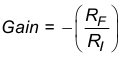Given that the TPA6111A2 is a MOS amplifier, the input impedance is high. Consequently, input leakage currents are not generally a concern, although noise in the circuit increases as the value of RF increases. In addition, a certain range of RF values is required for proper start-up operation of the amplifier. Taken together, TI recommends that the effective impedance seen by the inverting node of the amplifier be set between 5 kΩ and 20 kΩ. The effective impedance is calculated in Equation 2.

Equation 2.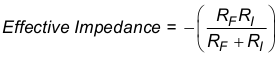As an example, consider an input resistance of 20 kΩ and a feedback resistor of 20 kΩ. The gain of the amplifier would be –1 and the effective impedance at the inverting terminal would be 10 kΩ, which is within the recommended range.

For high-performance applications, metal film resistors are recommended because they tend to have lower noise levels than carbon resistors. For values of RF above 50 kΩ, the amplifier tends to become unstable due to a pole formed from RF and the inherent input capacitance of the MOS input structure. For this reason, a small compensation capacitor of approximately 5 pF must be placed in parallel with RF. In effect, this creates a low-pass filter network with the cutoff frequency defined in Equation 3.

Equation 3.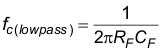For example, if RF is 100 kΩ and CF is 5 pF, then fc(lowpass) is 318 kHz, which is well outside the audio range.

#### 10.2.2.2 Input Capacitor, Ci

In the typical application, input capacitor CI is required to allow the amplifier to bias the input signal to the proper DC level for optimum operation. In this case, Ci and RI form a high-pass filter with the corner frequency determined in Equation 4.

Equation 4.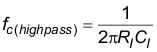The value of CI is important to consider, as it directly affects the bass (low-frequency) performance of the circuit. Consider the example where RI is 20 kΩ and the specification calls for a flat bass response down to 20 Hz. Equation 4 is reconfigured as Equation 5.

Equation 5.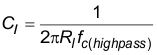In this example, CI is 0.40 µF, so TI recommends choosing a value in the range of 0.47 µF to 1 µF. A further consideration for this capacitor is the leakage path from the input source through the input network (RI, CI) and the feedback resistor (RF) to the load. This leakage current creates a DC offset voltage at the input to the amplifier that reduces useful headroom, especially in high-gain applications (> 10). For this reason a low-leakage tantalum or ceramic capacitor is the best choice. When polarized capacitors are used, the positive side of the capacitor must face the amplifier input in most applications, as the DC level there is held at VDD/2, which is likely higher than the source DC level.

NOTE

It is important to confirm the capacitor polarity in the application.

#### 10.2.2.3 Power Supply Decoupling, C(S)

The TPA6111A2 is a high-performance CMOS audio amplifier that requires adequate power supply decoupling to ensure that the output total harmonic distortion (THD) is as low as possible. Power supply decoupling also prevents oscillations for long lead lengths between the amplifier and the speaker. The optimum decoupling is achieved by using two capacitors of different types that target different types of noise on the power supply leads. For higher frequency transients, spikes, or digital hash on the line, a good low equivalent-series-resistance (ESR) ceramic capacitor, typically 0.1 µF, placed as close as possible to the device VDD lead, works best. For filtering lower frequency noise signals, a larger aluminum electrolytic capacitor of 10 µF or greater placed near the power amplifier is recommended.

#### 10.2.2.4 Midrail Bypass Capacitor, C(BYP)

The midrail bypass capacitor, C(BYP), serves several important functions. During start-up, C(BYP) determines the rate at which the amplifier starts up. This helps to push the start-up pop noise into the subaudible range (so low it cannot be heard). The second function is to reduce noise produced by the power supply caused by coupling into the output drive signal. This noise is from the midrail generation circuit internal to the amplifier. The capacitor is fed from a 230-kΩ source inside the amplifier. To keep the start-up pop as low as possible, the relationship shown in Equation 6 must be maintained.

Equation 6.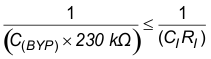As an example, consider a circuit where C(BYP) is 1 µF, CI is 1 µF, and RI is 20 kΩ. Inserting these values into Equation 6 results in: 6.25 ≤ 50 which satisfies the rule. Recommended values for bypass capacitor C(BYP) are 0.1-µF to 1-µF, ceramic or tantalum low-ESR, for the best THD and noise performance.

#### 10.2.2.5 Output Coupling Capacitor, C(C)

In the typical single-supply single-ended (SE) configuration, an output coupling capacitor (CC) is required to block the DC bias at the output of the amplifier, thus preventing DC currents in the load. As with the input coupling capacitor, the output coupling capacitor and impedance of the load form a high-pass filter governed by Equation 7.

Equation 7.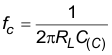The main disadvantage, from a performance standpoint, is that the typically small load impedances drive the low-frequency corner higher. Large values of C(C) are required to pass low frequencies into the load. Consider the example where a C(C) of 68 µF is chosen and loads vary from 32 Ω to 47 kΩ. Table 3 summarizes the frequency response characteristics of each configuration.

### Table 3. Common Load Impedances vs Low Frequency Output Characteristics in SE Mode

RL CC LOWEST FREQUENCY
32 Ω 68 µF  73 Hz
10,000 Ω 68 µF 0.23 Hz
47,000 Ω 68 µF 0.05 Hz

As Table 3 indicates, headphone response is adequate and drive into line level inputs (a home stereo for example) is good.

The output coupling capacitor required in single-supply SE mode also places additional constraints on the selection of other components in the amplifier circuit. With the rules described earlier still valid, add the following relationship in Equation 8:

Equation 8.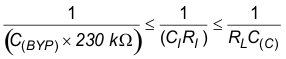#### 10.2.2.6 Using Low-ESR Capacitors

Low-ESR capacitors are recommended throughout this application. A real capacitor can be modeled simply as a resistor in series with an ideal capacitor. The voltage drop across this resistor minimizes the beneficial effects of the capacitor in the circuit. The lower the equivalent value of this resistance, the more the real capacitor behaves like an ideal capacitor.

#### 10.2.3 Application Curves

The characteristics of this design are shown in Table 4 from the Typical Characteristics section.

### Table 4. Table of Graphs

FIGURE
THD+N Total harmonic distortion plus noise vs Frequency Figure 11
vs Output power Figure 12